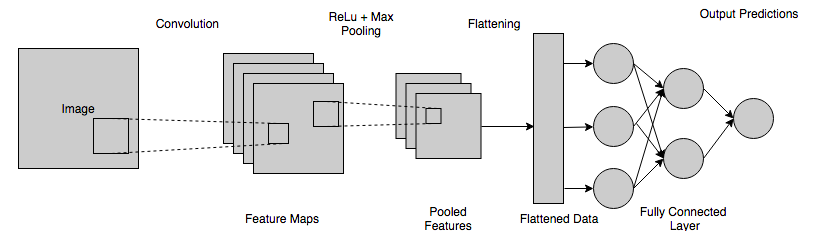/  Deep Learning Interview questions and answers   /  What is Convolutional Neural Network? What are all the layers used in it?## What is Convolutional Neural Network? What are all the layers used in it?

Ans: A Convolutional Neural Network is a Deep Learning algorithm which can take image as an input, assign weights and biases to various objects in the image and be able to differentiate one from the other. The pre-processing required in a ConvNet is much lower as compared to other classification algorithms.

Convolutional Neural Network architecture is somewhat similar to connectivity pattern of neurons in Human Brain and was inspired by organization of Visual Cortex.

### Layers used in Convolutional Neural Network

Convolution Layer:

The element which is involved in carrying out the convolution operation in the first part of a Convolutional Layer in Convolutional Neural Network is called the Kernel/Filter.  The Kernel has the same depth as that of the input image. Matrix Multiplication is performed between Kn and in stack ([K1, I1]; [K2, I2]; [K3, I3]) and all the results are summed with the bias to give us a squashed one-depth channel Convoluted Feature Output. The objective of the Convolution Operation is to extract the high-level features such as edges from the input image. ConvNets need not be limited to only one Convolutional Layer. Conventionally, the first ConvLayer is responsible for capturing the Low-Level features such as edges, color, gradient orientation, etc. With addition of the layers, the architecture adapts to the High-Level features as well, gives us a network, which has the good understanding of images in the dataset.Pooling Layer:

Similar to the Convolutional Layer, the Pooling layer is responsible for decreasing the spatial size of the Convolved Feature of the image. This is to reduce the computational power required to process the data through dimensionality reduction. Moreover, it is useful for extracting dominant features which are rotational and positional invariant which maintains the process of effectively training of the model.

There are two types of Pooling, Max Pooling and Average Pooling. Max Pooling yields the maximum value from the portion of the image enclosed by the Kernel. On the other hand, Average Pooling gives the average of all the values from the portion of the image covered by the Kernel.Fully connected Layer:

Fully-Connected layer is a cheap way of learning non-linear combinations of the high-level features as represented by the output of the convolutional layer. The Fully-Connected layer is learning a non-linear function in that space.